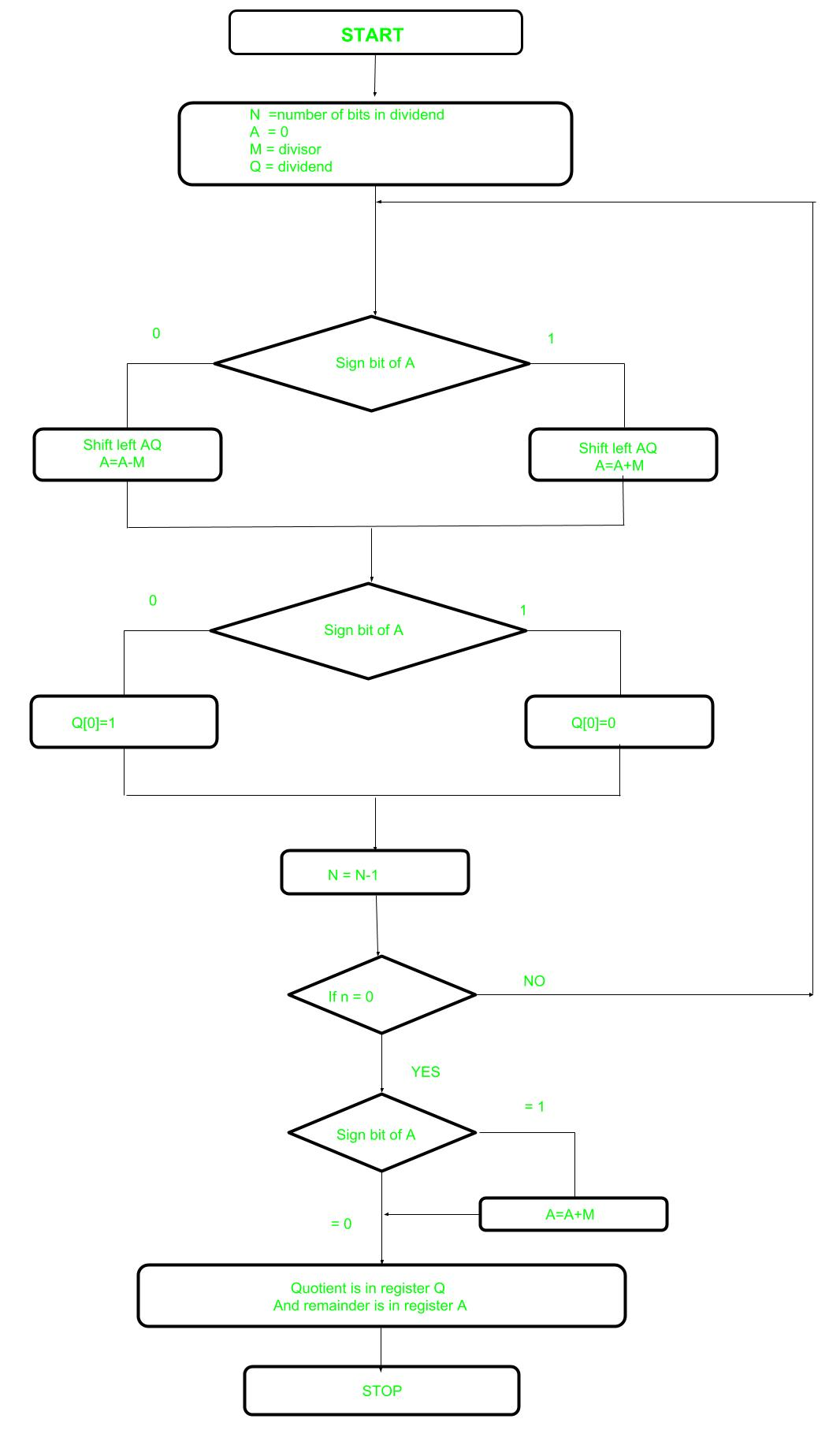# Non-Restoring Division For Unsigned Integer

In earlier post Restoring Division learned about restoring division. Now, here perform Non-Restoring division, it is less complex than the restoring one because simpler operation are involved i.e. addition and subtraction, also now restoring step is performed. In the method, rely on the sign bit of the register which initially contain zero named as A.

Here is the flow chart given below.Let’s pick the step involved:

• Step-1: First the registers are initialized with corresponding values (Q = Dividend, M = Divisor, A = 0, n = number of bits in dividend)
• Step-2: Check the sign bit of register A
• Step-3: If it is 1 shift left content of AQ and perform A = A+M, otherwise shift left AQ and perform A = A-M (means add 2’s complement of M to A and store it to A)
• Step-4: Again the sign bit of register A
• Step-5: If sign bit is 1 Q become 0 otherwise Q become 1 (Q means least significant bit of register Q)
• Step-6: Decrements value of N by 1
• Step-7: If N is not equal to zero go to Step 2 otherwise go to next step
• Step-8: If sign bit of A is 1 then perform A = A+M
• Step-9: Register Q contain quotient and A contain remainder
• Examples: Perform Non_Restoring Division for Unsigned Integer

```Dividend =11
Divisor  =3
-M =11101
```
N M A Q Action
4 00011 00000 1011 Start
00001 011_ Left shift AQ
11110 011_ A=A-M
3 11110 0110 Q=0
11100 110_ Left shift AQ
11111 110_ A=A+M
2 11111 1100 Q=0
11111 100_ Left Shift AQ
00010 100_ A=A+M
1 00010 1001 Q=1
00101 001_ Left Shift AQ
00010 001_ A=A-M
0 00010 0011 Q=1
```Quotient  = 3 (Q)
Remainder = 2 (A)
```My Personal Notes arrow_drop_upIf you like GeeksforGeeks and would like to contribute, you can also write an article using contribute.geeksforgeeks.org or mail your article to contribute@geeksforgeeks.org. See your article appearing on the GeeksforGeeks main page and help other Geeks.

Please Improve this article if you find anything incorrect by clicking on the "Improve Article" button below.

2

Please write to us at contribute@geeksforgeeks.org to report any issue with the above content.